top of page

### Power Cycles Analysis

This section provides free Power Cycles Analysis.

Power Cycles Analysis covers the following areas:
Carnot Cycle
Brayton Cycle -- Power Application
Brayton Cycle -- Propulsion Application
Otto Cycle
Diesel Cycle

Carnot Cycle

Introduction

This section provides a Carnot Cycle analysis when the working fluid is air.

Analysis

In the presented Carnot Cycle analysis, only air is considered as the working fluid behaving as a perfect gas -- specific heat has a constant value.  Ideal gas state equation is valid -- pv = RT.

Air enters a compressor at point 1 and it exits the compressor at point 2.  Isentropic compression is considered with no entropy change.  Air enters a heat exchanger -- heat addition -- at point 2 and it exits the heat exchanger at point 3.  At a constant temperature, heat addition takes place.  Air enters a turbine at point 3 and it exits the turbine at point 4.  Isentropic expansion is considered with no entropy change.  Air enters a heat exchanger -- heat rejection -- at point 4 and it exits the heat exchanger at point 1.   At a constant temperature, heat rejection takes place.  It should be mentioned that air at point 1 enters the compressor and the cycle is repeated.

Figure 1 contains a Carnot Cycle schematic layout.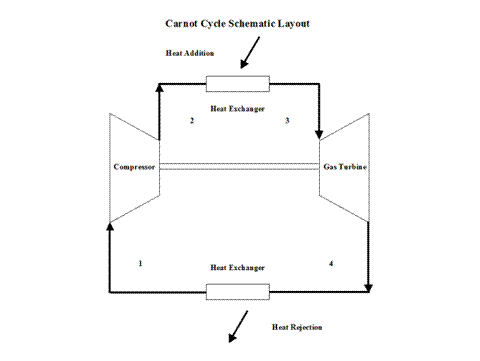Figure 1 - Carnot Cycle Schematic Layout

Figure 2 presents a Carnot Cycle entropy vs temperature diagram.Figure 2 - Carnot Cycle Temperature vs Entropy Diagram

Figure 3 presents the Carnot Cycle efficiency as a function of the heat addition temperature.  It should be noted that the inlet conditions are standard ambient conditions:  temperature of 298 [K] and absolute pressure of 1 [atm].Figure 3 - Carnot Cycle Efficiency vs Heat Addition Temperature

Figure 4 presents the Carnot Cycle efficiency as a function of the heat rejection temperature.  It should be noted that the turbine inlet temperature is at 800 [K].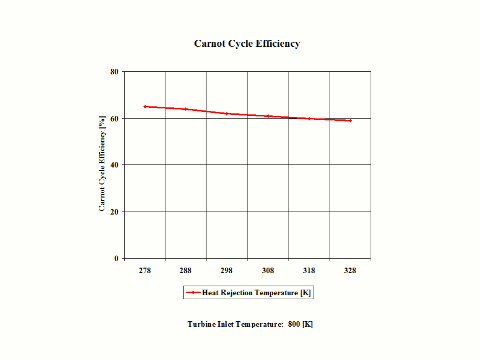Figure 4 - Carnot Cycle Efficiency vs Heat Rejection Temperature

One can notice that the Carnot Cycle efficiency increases with an increase in the heat addition temperature when the heat rejection temperature does not change at all.  One can notice that the Carnot Cycle efficiency decreases with an increase in the heat rejection temperature when the heat addition temperature does not change at all.

Assumptions

Working fluid is air.  There is no friction.  Compression and expansion are isentropic -- there is no entropy change.  During heat addition and heat rejection, the air temperature does not change.  Ideal gas state equation is valid -- pv = RT.  Air behaves as a perfect gas -- specific heat has a constant value.

Governing Equations

T2/T1 = (p2/p1)^(ϰ-1)/ϰ

T3/T4 = (p3/p4)^(ϰ-1)/ϰ
ϰ = cp/cv
c
p - cv = R
pv = RT
efficiency = 1 - T
1/T2
efficiency = 1 - T
R/TA

Input Data

T1 = 298 [K]
p
1 = 1 [atm]
R = 0.2867 [kJ/kg*K]
c
p = 1.004 [kJ/kg*K]
ϰ = 1.4 [/]

Results

Carnot Cycle Efficiency vs Heat Addition Temperature
Heat Rejection Temperature = 298 [K]

Temperature
[K]

500

600

700

800

900

1,000

Carnot Cycle
Efficiency
[%]

40

50

57

62

66

70

Carnot Cycle Efficiency vs Heat Rejection Temperature
Heat Addition Temperature = 800 [K]

Heat Rejection
Temperature
[K]

278

288

298

308

318

328

Carnot Cycle
Efficiency
[%]

65

64

62

61

60

59

Conclusions

The Carnot Cycle efficiency increases with an increase in the heat addition temperature when the heat rejection temperature does not change at all.   Furthermore, the Carnot Cycle efficiency decreases with an increase in the heat rejection temperature when the heat addition temperature does not change at all.  The Carnot Cycle efficiency is not dependent on the working fluid properties.

References

JANAF Thermochemical Data - Tables, 1970

Brayton Cycle -- Power Application

Introduction

This section provides a Brayton Cycle analysis when the working fluid is air.

Analysis

In the presented Brayton Cycle analysis, only air is considered as the working fluid behaving as a perfect gas -- specific heat has a constant value.  Ideal gas state equation is valid -- pv = RT.

A gas turbine is a heat engine that uses a high temperature, high pressure gas as the working fluid.  Combustion of a fuel in air is usually used to produce the needed temperatures and pressures in the gas turbine, which is why gas turbines are often referred to as combustion turbines.  Expansion of the high temperature, high pressure working fluid takes place in the gas turbine.  The gas turbine shaft rotation drives an electric generator and a compressor for the working fluid, air, used in the gas turbine combustor.  Many gas turbines also use a heat exchanger called a recouperator to impart turbine exhaust heat into the combustor's air/fuel mixture.  Gas turbines produce high quality heat that can be used to generate steam for combined heat and power and combined-cycle applications, significantly enhancing efficiency.

The initial state of the working fluid, air, is assumed to be at point 1 at the atmospheric conditions, 298 [K] temperature and 1 [atm] pressure.  Air is compressed, isentropically, along line 1-2 by a compressor and enters a combustor.  At a constant pressure, combustion takes place (fuel is added to the combustor and the air temperature raises) and/or heat gets added to air.  High temperature air exits the combustor at point 3.  Then air enters a gas turbine where an isentropic expansion occurs, producing power.  Air exits the gas turbine at point 4.  It should be mentioned that air at point 1 enters the compressor and the cycle is repeated.

A schematic layout of the ideal, open Brayton Cycle is shown in Figure 1.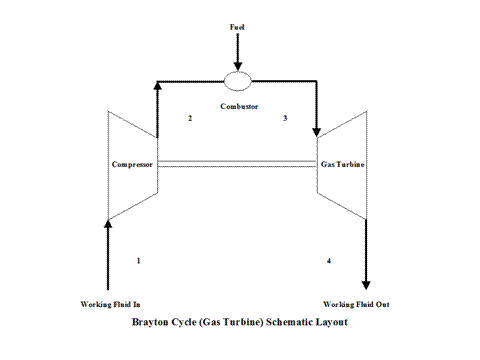Figure 1 - Brayton Cycle Schematic Layout

Figure 2 presents a Brayton Cycle temperature vs entropy diagram.Figure 2 - Brayton Cycle Temperature vs Entropy Diagram

It should be pointed out that this material deals with the open Brayton Cycle.  The way how the T - s diagram is presented, it describes a closed Brayton Cycle -- this would require a heat exchanger after point 4 where the working fluid would be cooled down to point 1 and the cycle repeats.  Therefore, the T - s diagram is presented as a closed Brayton Cycle to allow easier understanding and derivation of the Brayton Cycle thermal efficiency -- heat addition and heat rejection.

The gas turbine and compressor are connected by shaft so the considerable amount of work done on the gas turbine is used to power the compressor.
It can be noticed from the T - s diagram that the work done on the gas turbine is greater than the work necessary to power the compressor -- constant pressure lines in the T - s diagram diverge by going to the right side (entropy wise).

Figure 3 presents the Brayton Cycle efficiency as a function of the compression  ratio.  It should be noted that the inlet conditions are standard ambient conditions:  temperature of 298 [K] and absolute pressure of 1 [atm]. me. It's easy.Figure 3 - Brayton Cycle Efficiency

Here, two general performance trends are considered.  First, impact of the gas turbine inlet temperature and compression ratio values on the Brayton Cycle specific power output and second, impact of the working mass flow rate for a fixed gas turbine inlet temperature on the Brayton Cycle power output.

Figure 4 presents the results of the first performance trend, while Figure 5 presents the results of the second trend.Figure 4 - Brayton Cycle Specific Power Output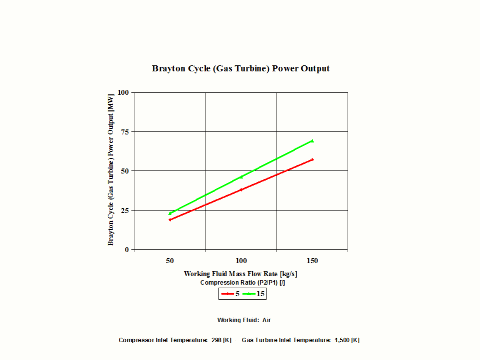Figure 5 - Brayton Cycle Power Output

One can notice that the Brayton Cycle efficiency increases with an increase in the compression ratio values.  One can notice that the Brayton Cycle specific power output increases with an increase in the gas turbine inlet temperature.  Furthermore, the increase is greater for the higher compression ratio values.

One can notice that the Brayton Cycle power output increases with an increase in the working fluid mass flow rate for a fixed gas turbine inlet temperature.  The increase is greater for the higher compression ratio values.

Assumptions

Working fluid is air.  There is no friction.  Compression and expansion processes are reversible and adiabatic -- isentropic.  Ideal gas state equation is valid -- pv = RT.  Air behaves as a perfect gas --specific heat has a constant value.

Governing Equations

T2/T1 = (p2/p1)^(ϰ-1)/ϰ
p
2/p1 = (T2/T1)^
ϰ/(ϰ-1)
T
3/T4 = (p3/p4)^(
ϰ-1)/ϰ
p
3/p4 = (T3/T4)^
ϰ/(ϰ-1)
ϰ = cp/cv
cp - cv = R
pv = RT
w = q
h - q
qh = cp(T3 - T2
q
l = cp(T4 - T1
w = c
p(T3 - T2) - cp(T4 - T1
W = (c
p(T3 - T2) - cp(T4 - T1))m
efficiency = 1 - 1/r
p^(
ϰ-1)/ϰ
r
p = p2/p1

Input Data

T1 = 298 [K]

p1 = 1 [atm]
T
3 = 900, 1,200 and 1,500 [K]

p3 = 5, 10, 15, 20 and 25 [atm]
R = 0.2867 [kJ/kg*K]
c
p = 1.004 [kJ/kg*K]

ϰ = 1.4 [/]

m = 50, 100 and 150 [kg/s]

Results

Brayton Cycle Efficiency vs Compression Ratio

Compression
Ratio
[/]

5

10

15

20

25

Brayton Cycle
Efficiency
[%]

36.92

48.22

53.87

57.53

60.16

Compression
Ratio
[/]

5

10

15

20

25

Brayton Cycle
Efficiency
[%]

36.92

48.22

53.87

57.53

60.16

Specific Power Output vs Compression Ratio
for a few Gas Turbine Inlet Temperature Values

Specific Power Output
[kW/kg/s]

Compression Ratio
[/]

5

15

Gas Turbine Inlet
Temperature
[K]

900

159

137

1,200

270

300

1,500

381

462

Power Output vs Compression Ratio
for a few Mass Flow Rates
Gas Turbine Inlet Temperature = 1,500 [K]

Power Output
[MW]

Compression Ratio
[/]

5

15

Mass Flow Rate
[kg/s]

50

19.1

23.1

100

38.1

46.2

150

57.2

69.3

Conclusions

The Brayton Cycle efficiency depends on the compression ratio and working fluid properties.  The efficiency increases with an increase in the compression ratio values.  Also, the efficiency increases with the higher value for kappa, ϰ, which is a ratio of gas specific heat values (cp/cv).

The Brayton Cycle specific power output increases with an increase in the gas turbine inlet temperature.  Furthermore, the increase is greater for the higher compression ratio values.  The Brayton Cycle power output increases with an increase in the working fluid mass flow rate for a fixed gas turbine inlet temperature.  The increase is greater for the higher compression ratio values.

References

JANAF Thermochemical Data - Tables, 1970

Brayton Cycle -- Propulsion Application

Introduction

This section provides a Brayton Cycle analysis when the working fluid is air.

Analysis

In the presented Brayton Cycle analysis, only air is considered as the working fluid behaving as a perfect gas -- specific heat has a constant value. Ideal gas state equation is valid -- pv = RT.

A gas turbine is a heat engine that uses a high temperature, high pressure gas as the working fluid.  Combustion of a fuel in air is usually used to produce the needed temperatures and pressures in the gas turbine, which is why gas turbines are often referred to as combustion turbines. Expansion of the high temperature, high pressure working fluid takes place in the gas turbine.  The gas turbine shaft rotation drives an electric generator and a compressor for the working fluid, air, used in the gas turbine combustor.  Many gas turbines also use a heat exchanger called a recouperator to impart turbine exhaust heat into the combustor's air/fuel mixture.  Gas turbines produce high quality heat that can be used to generate steam for combined heat and power and combined-cycle applications, significantly enhancing efficiency.

The initial state of the working fluid, air, is assumed to be at point 1 at the atmospheric conditions, 298 [K] temperature and 1 [atm] pressure.  Air is compressed, isentropically, along line 1-2 by a compressor and enters a combustor.  At a constant pressure, combustion takes place (fuel is added to the combustor and the air temperature raises) and/or heat gets added to air.  High temperature air exits the combustor at point 3.  Then air enters a gas turbine where an isentropic expansion occurs, producing power.  Air exits the gas turbine at point 4.  It should be mentioned that air at point 1 enters the compressor and the cycle is repeated.

Figure 1 presents a Brayton Cycle schematic layout.

Figure 1 - Brayton Cycle Schematic Layout

Figure 2 presents a Brayton Cycle temperature vs entropy diagram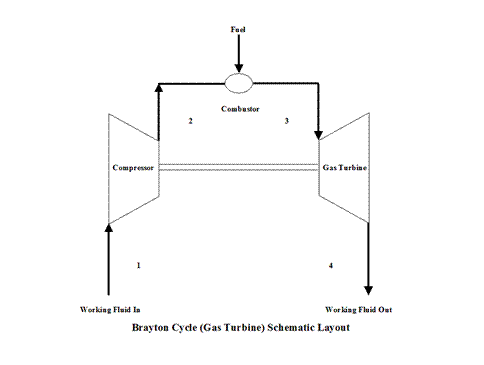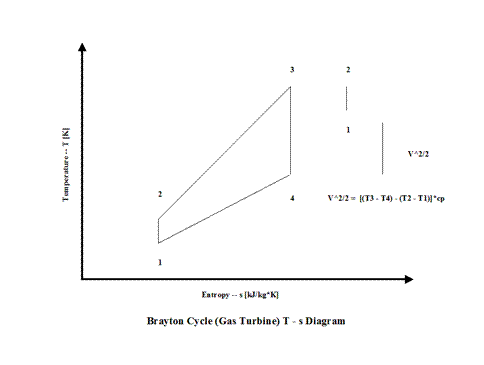Figure 2 - Brayton Cycle Temperature vs Entropy Diagram

In order to keep the scope of thrust analysis simple, air exiting turbine expands to the atmospheric conditions -- exit pressure is equal to the ambient pressure (p1= p4 ).

It should be pointed out that this material deals with the open Brayton Cycle.  The way how the T - s diagram is presented, it describes a closed Brayton Cycle -- this would require a heat exchanger after point 4 where the working fluid would be cooled down to point 1 and the cycle repeats. Therefore, the T - s diagram is presented as a closed Brayton Cycle to allow easier understanding and derivation of the Brayton Cycle thermal efficiency -- heat addition and heat rejection.

The gas turbine and compressor are connected by shaft so the considerable amount of work done on the gas turbine is used to power the compressor.

Propulsion is provided by the difference of gas turbine expansion minus the compressor power requirements.

It can be noticed from the T - s diagram that the work done on the gas turbine is greater than the work necessary to power the compressor -- constant pressure lines in the T - s diagram diverge by going to the right side (entropy wise).

Figure 3 presents the Brayton Cycle efficiency as a function of the compression ratio.  It should be noted that the inlet conditions are standard ambient conditions: temperature of 298 [K] and absolute pressure of 1 [atm].Figure 3 - Brayton Cycle Efficiency

Here, two general performance trends are considered.  First, impact of the gas turbine inlet temperature and compression ratio on the Brayton Cycle specific propulsion output and second, impact of the working mass flow rate for a fixed gas turbine inlet temperature on the Brayton Cycle propulsion output.

Figure 4 presents the results of the first performance trend, while Figure 5 presents the results of the second trend.Figure 4 - Brayton Cycle Specific Propulsion OutputFigure 5 - Brayton Cycle Propulsion Output

One can notice that the Brayton Cycle efficiency increases with an increase in the compression ratio values.  One can notice that the Brayton Cycle specific power output increases with an increase in the gas turbine inlet temperature.  Furthermore, the increase is greater for the higher compression ratio values.

One can notice that the Brayton Cycle propulsion output increases with an increase in the working fluid mass flow rate for a fixed gas turbine inlet temperature. The increase is greater for the higher compression ratio values.

Assumptions

Working fluid is air.  There is no fricion.  Compression and expansion processes are reversible and adiabatic -- isentropic.  Ideal gas state equation is valid -- pv = RT.  Air behaves as a perfect gas --specific heat has a constant value.

Governing Equations

T2/T1 = (p2/p1)^(ϰ-1)/ϰ
p
2/p1 = (T2/T1)^
ϰ/(ϰ-1)
T
3/T4 = (p3/p4)^(
ϰ-1)/ϰ
p
3/p4 = (T3/T4)^
ϰ/(ϰ-1)
ϰ = cp/cv
cp - cv = R
pv = RT
w = q
h - ql
q
h = cp(T3 - T2
q
l = cp(T4 - T1)
w = c
p(T3 - T2) - cp(T4 - T1
W = (c
p(T3 - T2) - cp(T4 - T1))m
efficiency = 1 - 1/r
p^(
ϰ-1)/ϰ
r
p = p2/p1
v^2/2 = c
p((T3 - T2) - (T4 - T1))
v = (2c
p((T3 - T2) - (T4 - T1)))^1/2
Thrust = vm

Input Data

T1 = 298 [K]

p1 = 1 [atm]
T
3 = 900, 1,200 and 1,500 [K]

p3 = 5, 10, 15, 20 and 25 [atm]
R = 0.2867 [kJ/kg*K]
c
p = 1.004 [kJ/kg*K]

ϰ = 1.4 [/]

m = 50, 100 and 150 [kg/s]

Results

Brayton Cycle Efficiency vs Compression Ratio

Compression
Ratio
[/]

5

10

15

20

25

Brayton Cycle
Efficiency
[%]

36.92

48.22

53.87

57.53

60.16

Compression
Ratio
[/]

5

10

15

20

25

Brayton Cycle
Efficiency
[%]

36.92

48.22

53.87

57.53

60.16

Specific Propulsion Output vs Compression Ratio
for a few Gas Turbine Inlet Temperature Values

Specific
Propulsion

[N/kg/s]

Compression Ratio
[/]

5

15

Gas Turbine Inlet
Temperature
[K]

900

563

524

1,200

734

774

1,500

872

961

Propulsion Output vs Compression Ratio
for a few Mass Flow Rates
Gas Turbine Inlet Temperature = 1,500 [K]

Propulsion Output
[kN]

Compression Ratio
[/]

5

15

Mass Flow Rate
[kg/s]

50

43.61

48.06

100

87.23

96.11

150

130.85

144.17

Conclusions

The Brayton Cycle efficiency depends on the compression ratio and working fluid properties.  The efficiency increases with an increase in the compression ratio values.  Also, the efficiency increases with the higher value for
ϰ, which is a ratio of gas specific heat values (cp/cv).

The Brayton Cycle specific propulsion increases with an increase in the gas turbine inlet temperature.  Furthermore, the increase is greater for the higher compression ratio values.  The Brayton Cycle propulsion increases with an increase in the working fluid mass flow rate for a fixed gas turbine inlet temperature.  The increase is greater for the higher compression ratio values.

References

JANAF Thermochemical Data - Tables, 1970

Otto Cycle

Introduction

This section provides an Otto Cycle analysis when the working fluid is air.

Analysis

In the presented Otto Cycle analysis, only air is considered as the working fluid behaving as a perfect gas -- specific heat has a constant value.  Ideal gas state equation is valid -- pv = RT.

Air enters a cylinder at point 1 when compression starts and it ends at point 2.  Isentropic compression is considered with no entropy change.  Heat addition starts at point 2 and it ends at point 3.  At a constant volume, air gets heated and the working fluid temperature raises.  Expansion starts at point 3 and it ends at point 4.  Isentropic expansion is considered with no entropy change.  Air heat rejection starts at point 4 and it ends at point 1.   At a constant volume, air gets cooled and the working fluid temperature decreases.  It should be mentioned that air at point 1 enters the compression process again and the cycle is repeated.

Figure 1 contains an Otto Cycle pressure vs volume diagram.Figure 1 - Otto Cycle Pressure vs Volume Diagram

Figure 2 presents an Otto Cycle temperature vs entropy diagram.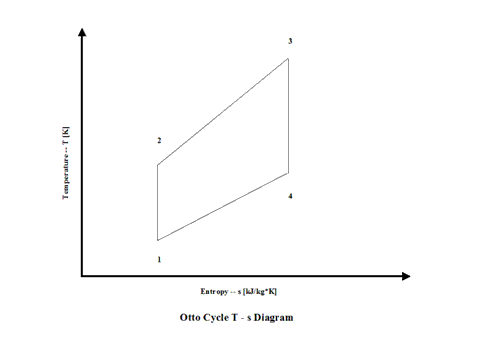Figure 2 - Otto Cycle Temperature vs Entropy Diagram

Figure 3 presents the Otto Cycle efficiency as a function of the compression ratio.  It should be noted that the inlet conditions are standard ambient conditions:  temperature of 298 [K] and absolute pressure of 1 [atm].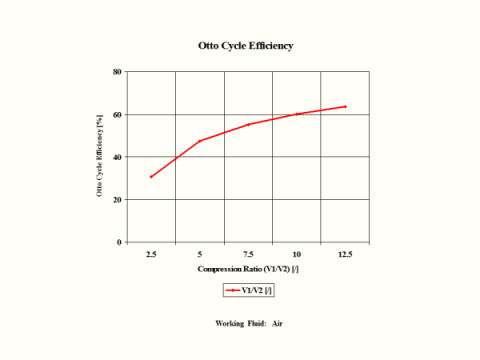Figure 3 - Otto Cycle Efficiency

Figure 4 presents the Otto Cycle power output as a function of the combustion temperature and compression ratio.  It should be noted that the number of revolutions is 60 [1/s] for given geometry of the four cylinder and four stroke Otto engine.Figure 4 - Otto Cycle Power  Output

One can notice that the Otto Cycle efficiency increases with an increase in the compression ratio values.  One can notice that the Otto Cycle power output increases with an increase in the combustion temperature.  The Otto Cycle power output is greater for the higher compression ratio values.

Assumptions

Working fluid is air.  There is no friction.  Compression and expansion are isentropic -- there is no entropy change.  Ideal gas state equation is valid -- pv = RT.  Air behaves as a perfect gas -- specific heat has a constant value.

Governing Equations

T2/T1 = (V1/V2)^(ϰ-1)
V
1/V2 = (T2/T1)^1/(
ϰ-1)

T3/T4 = (V4/V3)^(ϰ-1)
V
4/V3 = (T3/T4)^1/(
ϰ-1)
ϰ = cp/cv
cp - cv = R
pv = RT
w = q
h - ql
qh = cv(T3 - T2)
q
l = cv(T4 - T1)
w = c
v(T3 - T2) - cv(T4 - T1)
W = (c
v(T3 - T2) - cv(T4 - T1))m
efficiency = 1 - 1/compression ratio^(
ϰ-1)
compression ratio = V
1/V2

Input Data

T1 = 298 [K]
p
1 = 1 [atm]
T
3 =  1,200, 1,500 and 1,800 [K]

compression ratio = 2.5, 5, 7.5, 10 and 12.5 [/]
R = 0.2867 [kJ/kg*K]
c
p = 1.004 [kJ/kg*K]

ϰ = 1.4 [/]

Results

Otto Cycle Efficiency vs Compression Ratio

Compression
Ratio
[/]

2.5

5

7.5

10

12.5

Otto Cycle
Efficiency
[%]

30.7

47.5

55.3

60.2

63.6

Otto Cycle Power Output

Power Output
[kW]

Compression Ratio
[/]

5

15

Combustion
Temperature
[K]

1,200

167.1

151.2

1,500

246.4

251.7

1,800

325.6

352.2

Conclusions

The Otto Cycle efficiency increases with an increase in the compression ratio values.  Also, the Otto Cycle power output increases with an increase in the combustion temperature.  The Otto Cycle power output is greater for the higher compression ratio values.

References

JANAF Thermochemical Data - Tables, 1970

Diesel Cycle

Introduction

This section provides a Diesel Cycle analysis when the working fluid is air.

Analysis

In the presented Diesel Cycle analysis, only air is considered as the working fluid behaving as a perfect gas -- specific heat has a constant value.  Ideal gas state equation is valid -- pv = RT.

Air enters a cylinder at point 1 when compression starts and it ends at point 2.  Isentropic compression is considered with no entropy change.  Heat addition starts at point 2 and it ends at point 3.  At a constant pressure, air gets heated and the working fluid temperature raises.  Expansion starts at point 3 and it ends at point 4.  Isentropic expansion is considered with no entropy change.  Air heat rejection starts at point 4 and it ends at point 1.  At a constant volume, air gets cooled and the working fluid temperature decreases.  It should be mentioned that air at point 1 enters the compression process again and the cycle is repeated.

Figure 1 contains a Diesel Cycle pressure vs volume diagram.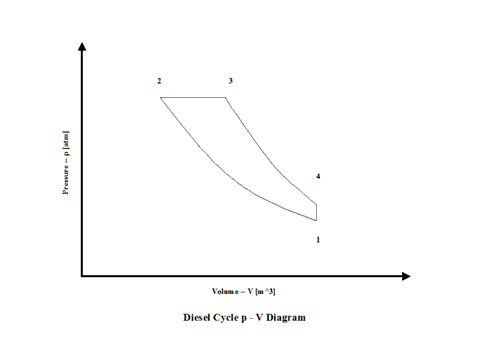Figure 1  -  Diesel Cycle Pressure vs Volume Diagram

Figure 2 presents a Diesel Cycle temperature vs entropy diagram.

igure 1 - Diesel Cycle Pressure vs Volume Diagram

Figure 2 presents a Diesel Cycle temperature vs entropy diagram.Figure 2 - Diesel Cycle Temperature vs Entropy Diagram

Figure 3 presents the Diesel Cycle efficiency as a function of the compression ratio and a cut off ratio.  It should be noted that the inlet conditions are standard ambient conditions:  temperature of 298 [K] and absolute pressure of 1 [atm].Figure 3 - Diesel Cycle Efficiency

Figure 4 presents the Diesel Cycle power output as a function of compression and combustion temperature values.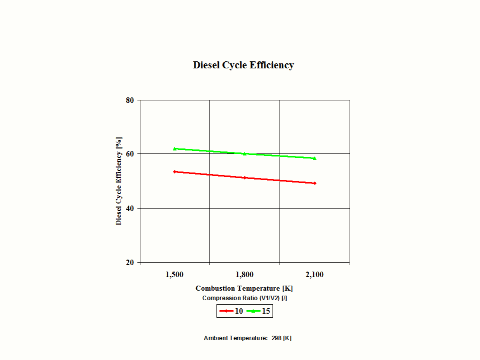Figure 4 - Diesel Cycle Power  Output

Figure 5 presents the Diesel Cycle cut off ratio as a function of the combustion temperature and compression ratio.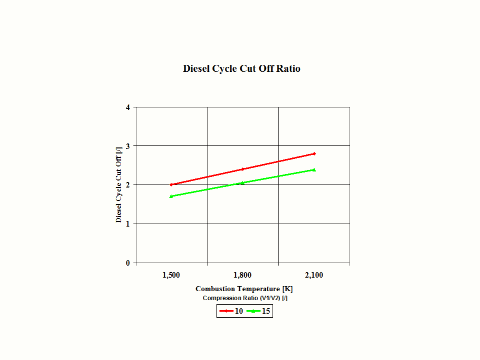Figure 5 - Diesel Cycle Cut Off Ratio

Figure 6 presents the Diesel Cycle power output as a function of the combustion temperature and compression ratio.  It should be noted that the number of revolutions is 60 [1/s] for given geometry of the four cylinder and four stroke Diesel engine.

Figure 5 - Diesel Cycle Cut Off Ratio

Figure 6 presents the Diesel Cycle power output as a function of the combustion temperature and compression ratio.  It should be noted that the number of revolutions is 60 [1/s] for given geometry of the four cylinder and four stroke Diesel engine.Figure 6 - Diesel Cycle Power Output

One can notice that the Diesel Cycle efficiency increases with an increase in the compression ratio and a decrease of the cut off ratio values.  One can notice that the Diesel Cycle power output increases with an increase in the compression ratio and combustion temperature values.

Assumptions

Working fluid is air.  There is no friction.  Compression and expansion are isentropic -- there is no entropy change.  Ideal gas state equation is valid -- pv = RT.  Air behaves as a perfect gas -- specific heat has a constant value.

Governing Equations

T2/T1 = (V1/V2)^(ϰ-1)
V
1/V2 = (T2/T1)^1/(
ϰ-1)

T3/T4 = (V4/V3)^(ϰ-1)
V
4/V3 = (T3/T4)^1/(
ϰ-1)
ϰ = cp/cv
cp - cv = R
pv = RT
w = q
h - ql
q
h = cp(T3 - T2
q
l = cv(T4 - T1
w = c
p(T3 - T2) - cv(T4 - T1
W = (c
p(T3 - T2) - cv(T4 - T1))m
efficiency = 1 - (cut off ratio^
ϰ - 1)/(ϰ(compression ratio^(ϰ-1))(cut off ratio - 1))
compression ratio = V
1/V2
cut off ratio = V
3/V2

Input Data

T1 = 298 [K]
p
1 = 1 [atm]

compression ratio = 7.5, 10, 12.5, 15 and 17.5 [/]

cut off ratio = 3 and 4 [/]
R = 0.2867 [kJ/kg*K]
c
p = 1.004 [kJ/kg*K]

ϰ = 1.4 [/]

Results

Diesel Cycle Efficiency

Diesel Cycle Efficiency
[%]

Cut Off Ratio
[/]

3

4

7.5

41.69

36.57

Compression
Ratio
[/]

10

48.03

43.4

12.5

52.46

48.29

15

55.81

51.93

17.5

58.45

54.80

Diesel Cycle Efficiency

Diesel Cycle

Efficiency
[%]

Compression Ratio
[/]

10

15

Combustion
Temperature
[K]

1,500

53.39

61.94

1,800

51.12

60.12

2,100

49.20

58.49

Diesel Cycle Cut Off Ratio

Cut Off Ratio
[/]

Compression Ratio
[/]

10

15

Combustion
Temperature
[K]

1,500

2.00

1.70

1,800

2.40

2.05

2,100

2.80

2.39

Diesel Cycle Power Output

Power Output
[kW]

Compression Ratio
[/]

10

15

Combustion
Temperature
[K]

1,500

311

297

1,800

417

433

2,100

514

557

Conclusions

The Diesel Cycle efficiency increases with an increase in the compression ratio and a decrase in the cut off rati values.  Also, the Diesel Cycle power output increases with an increase in the compression ratio and combustion temperature values.

References

JANAF Thermochemical Data - Tables, 1970

bottom of page